2015-06-04 21:31:33 baidu_28563321 阅读数 1487
• ###### OpenCV级联分类器训练与使用实战教程

基于OpenCV新版本3.1.0详细讲述了HAAR与LBP级联分类器的基本原理与使用技巧，通过视频中人脸实时检测与眼睛跟踪例子演示了级联分类器在项目中的运用。通过OpenCV自带的训练工具实现样本数据训练生成自己的级联分类器文件，使用它实现自定义对象检测。

1579 人正在学习 去看看 贾志刚

```//离散Haar小波变换
/*
dst深度为IPL_DEPTH_32F
nLayer为变换尺度
*/
void HaarWavelet(IplImage* src, IplImage* dst, int nLayer);```
```//离散Haar小波变换
/*
dst深度为IPL_DEPTH_32F
nLayer为变换尺度
*/
void HaarWavelet(IplImage* src, IplImage* dst, int nLayer)
{
if (!dst)
{
return;
}
if (((dst->width >> nLayer) << nLayer != dst->width)
|| ((dst->height >> nLayer) << nLayer != dst->height)
|| (dst->depth != IPL_DEPTH_32F)
|| (src->nChannels != 1)
|| (dst->nChannels != 1)
|| (nLayer <= 0))
{
return;
}

int x, y;
int nWidth = dst->width;
int nHeight = dst->height;
int nHalfWidth = nWidth / 2;
int nHalfHeight = nHeight / 2;
//图像数据的起始地址
float* *pfData = (float**)(malloc(sizeof(float*) * nHeight));
//保存计算过程中用到的行列数据
float* pfRow = (float*)(malloc(sizeof(float) * nWidth));
float* pfColumn = (float*)(malloc(sizeof(float) * nHeight));
CvMat tmp;
//预先填充dst
cvZero(dst);
cvGetSubRect(dst, &tmp, cvRect(0, 0, src->width, src->height));
cvScale(src, &tmp, 1.0, 0);
//保存图像数据每行的起始地址
for (y = 0; y < nHeight; y++)
{
pfData[y] = (float*)(dst->imageData + y * dst->widthStep);
}

while (nLayer > 0)
{
//行变换
for (y = 0; y < nHeight; y++)
{
for (x = 0; x < nHalfWidth; x++)
{
pfRow[x] = (pfData[y][2 * x] + pfData[y][2 * x + 1]) / 2.0;
pfRow[x + nHalfWidth] = (pfData[y][2 * x] - pfData[y][2 * x + 1]) / 2.0;
}
for (x = 0; x < nWidth; x++)
{
pfData[y][x] = pfRow[x];
}
}
//列变换
for (x = 0; x < nWidth; x++)
{

for (y = 0; y < nHalfHeight; y++)
{
pfColumn[y] = (pfData[2 * y][x] + pfData[2 * y + 1][x]) / 2;
pfColumn[y + nHalfHeight] = (pfData[2 * y][x] - pfData[2 * y + 1][x]) / 2;
}
for (y = 0; y < nHeight; y++)
{
pfData[y][x] = pfColumn[y];
}
}
//一层变换后尺度的缩小
nLayer--;
nWidth = nHalfWidth;
nHeight = nHalfHeight;
nHalfWidth = nHalfWidth / 2;
nHalfHeight = nHalfHeight / 2;
}

//结果缩放以便与显示
double min,max;
cvMinMaxLoc(dst, &min, &max, NULL, NULL, NULL);
cvScale(dst, dst, 1.0/(max-min), 1.0*(-min)/(max-min));
}
```

2014-03-30 20:55:16 yu12345678900 阅读数 3060
• ###### OpenCV级联分类器训练与使用实战教程

基于OpenCV新版本3.1.0详细讲述了HAAR与LBP级联分类器的基本原理与使用技巧，通过视频中人脸实时检测与眼睛跟踪例子演示了级联分类器在项目中的运用。通过OpenCV自带的训练工具实现样本数据训练生成自己的级联分类器文件，使用它实现自定义对象检测。

1579 人正在学习 去看看 贾志刚

## matlab练习程序（图像Haar小波变换）

1.把小波w(t)和原函数f(t)的开始部分进行比较，计算系数C。系数C表示该部分函数与小波的相似程度。

2.把小波向右移k单位，得到小波w(t-k)，重复1。重复该部知道函数f结束.

3.扩展小波w(t)，得到小波w(t/2)，重复步骤1,2.

4.不断扩展小波，重复1,2,3.main.m

```clear all;
close all;
clc;

[m n]=size(img);

[LL LH HL HH]=haar_dwt2D(img);  %当然dwt2(img,'haar')是一样的，我只是想明白细节
img=[LL LH;HL HH];      %一层分解

imgn=zeros(m,n);
for i=0:m/2:m/2
for j=0:n/2:n/2
[LL LH HL HH]=haar_dwt2D(img(i+1:i+m/2,j+1:j+n/2)); %对一层分解后的四个图像分别再分解
imgn(i+1:i+m/2,j+1:j+n/2)=[LL LH;HL HH];
end
end

imshow(imgn)```

haar_dwt2D.m

```function [LL LH HL HH]=haar_dwt2D(img)
[m n]=size(img);
for i=1:m       %每一行进行分解
[L H]=haar_dwt(img(i,:));
img(i,:)=[L H];
end
for j=1:n       %每一列进行分解
[L H]=haar_dwt(img(:,j));
img(:,j)=[L H];
end
%本来分解不应该加mat2gray的，不过为了有好的显示效果就加上了
LL=mat2gray(img(1:m/2,1:n/2));          %行列都是低频
LH=mat2gray(img(1:m/2,n/2+1:n));        %行低频列高频
HL=mat2gray(img(m/2+1:m,1:n/2));        %行高频列低频
HH=mat2gray(img(m/2+1:m,n/2+1:n));      %行列都是高频

end```

haar_dwt.m

```function [L H]=haar_dwt(f)　　%显然，我没有做边界处理，图片最好是2^n*2^n型的
n=length(f);
n=n/2;
L=zeros(1,n);   %低频分量
H=zeros(1,n);   %高频分量
for i=1:n
L(i)=(f(2*i-1)+f(2*i))/sqrt(2);
H(i)=(f(2*i-1)-f(2*i))/sqrt(2);
end

end```

2019-07-26 20:42:44 Arthur_Holmes 阅读数 557
• ###### OpenCV级联分类器训练与使用实战教程

基于OpenCV新版本3.1.0详细讲述了HAAR与LBP级联分类器的基本原理与使用技巧，通过视频中人脸实时检测与眼睛跟踪例子演示了级联分类器在项目中的运用。通过OpenCV自带的训练工具实现样本数据训练生成自己的级联分类器文件，使用它实现自定义对象检测。

1579 人正在学习 去看看 贾志刚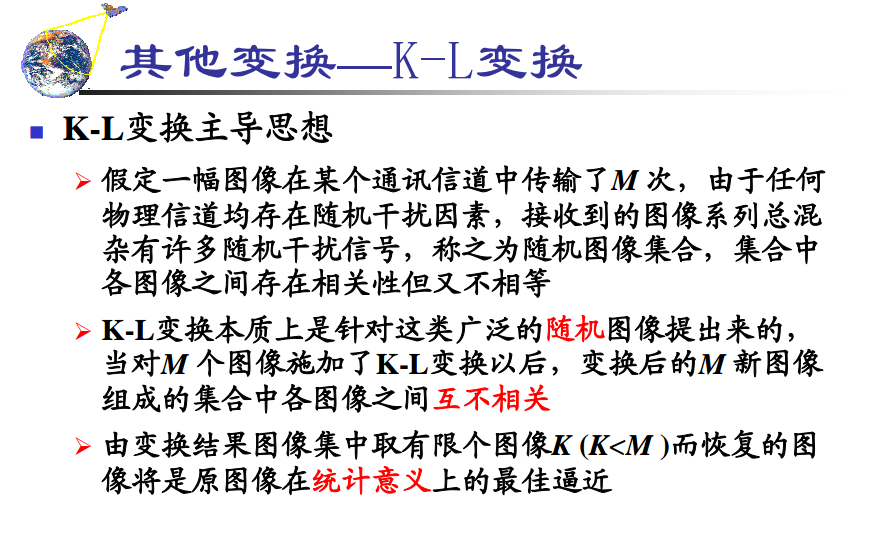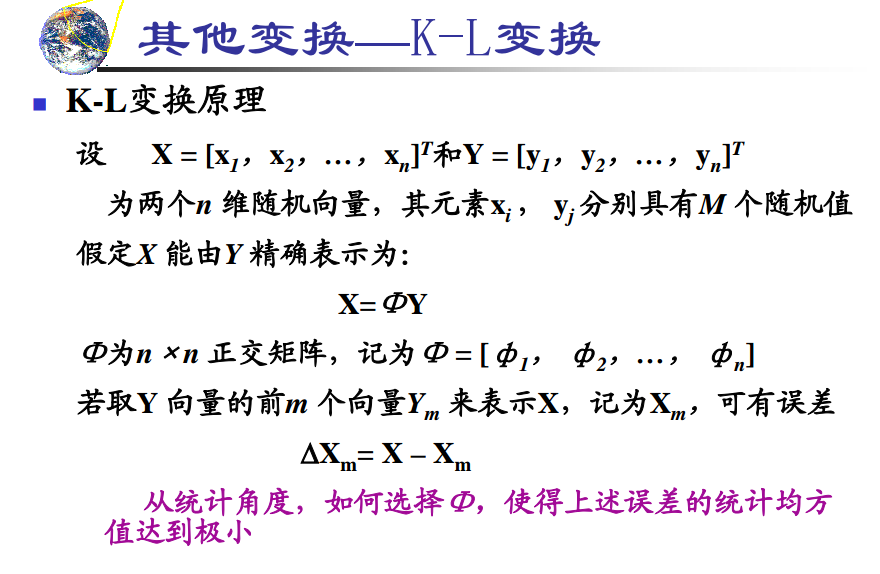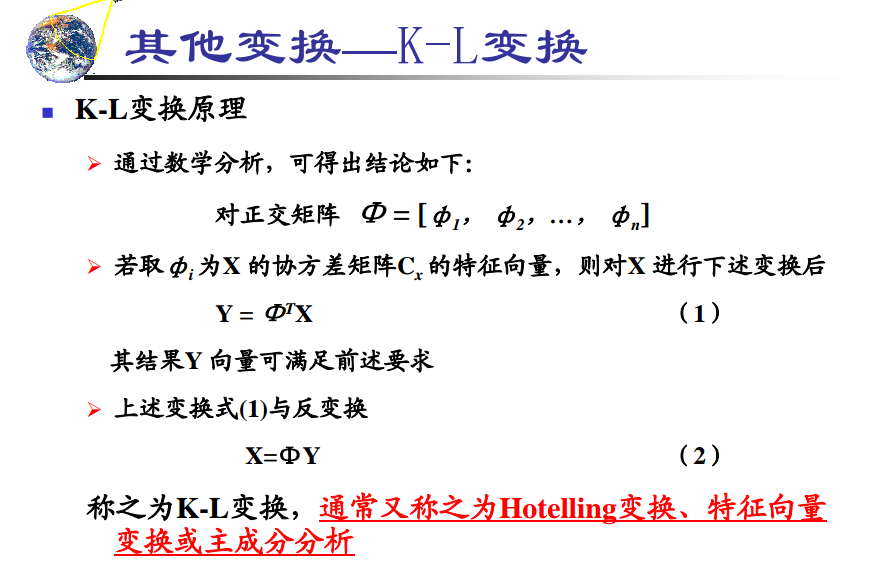https://zh.wikipedia.org/wiki/%E5%8D%8F%E6%96%B9%E5%B7%AE%E7%9F%A9%E9%98%B5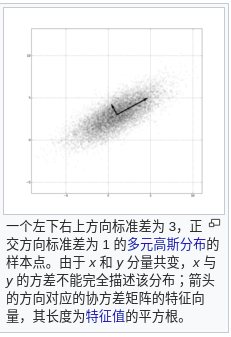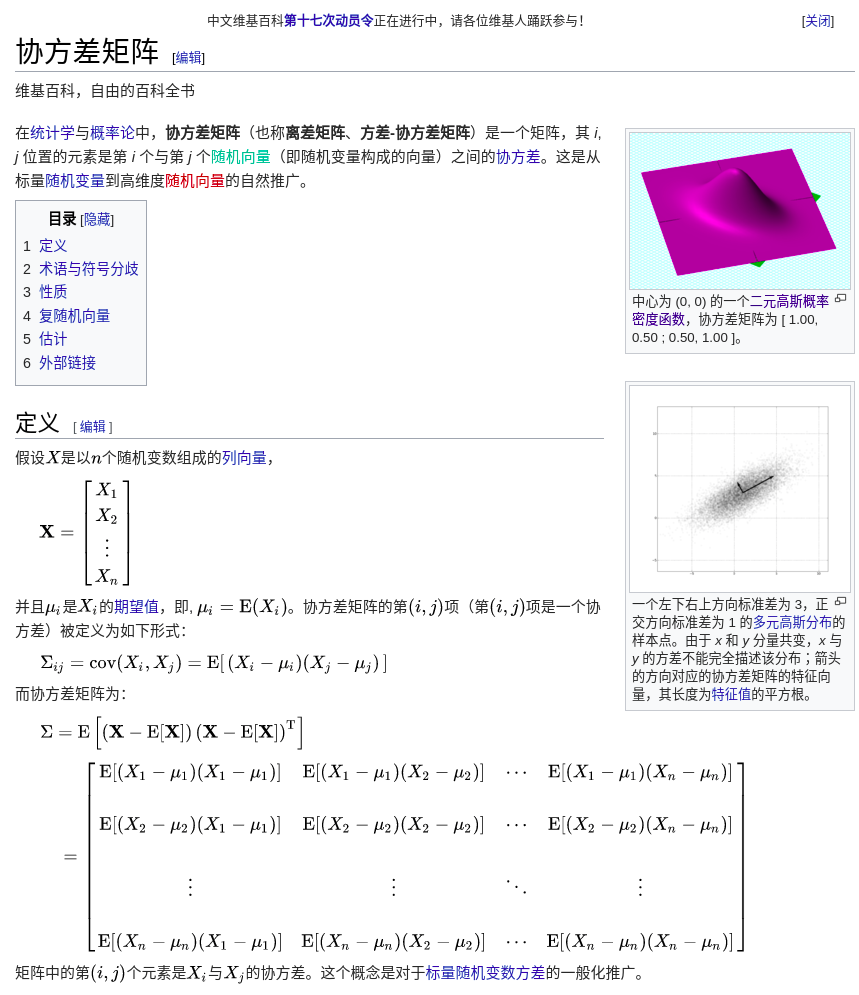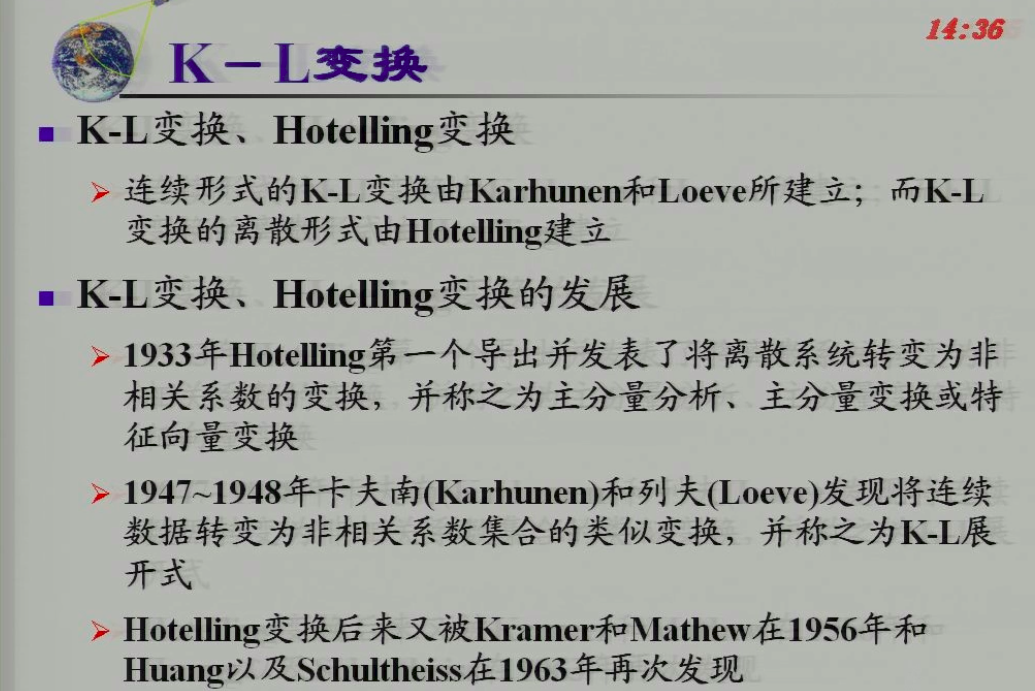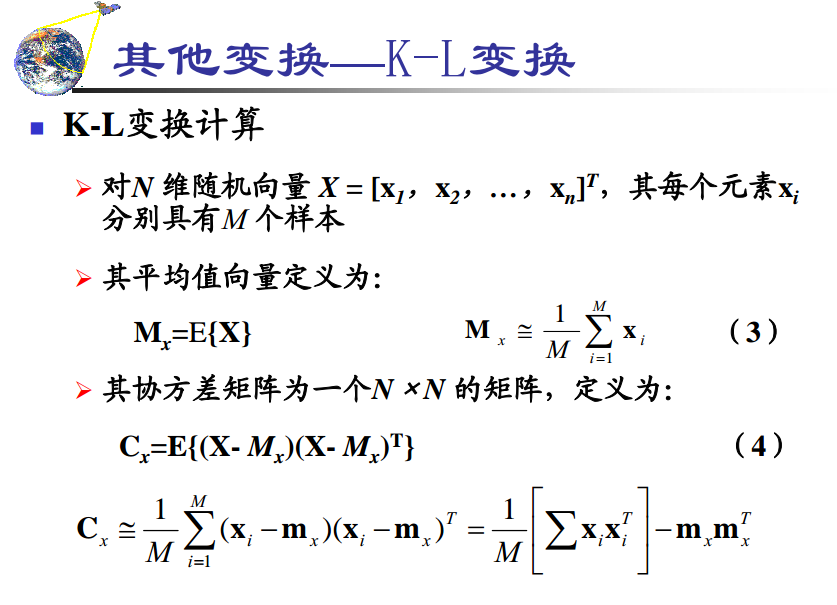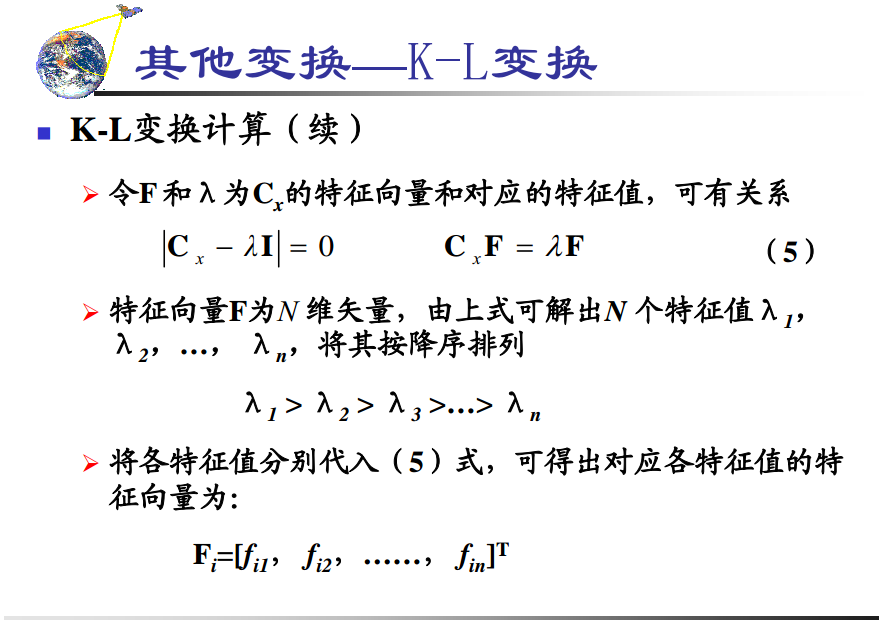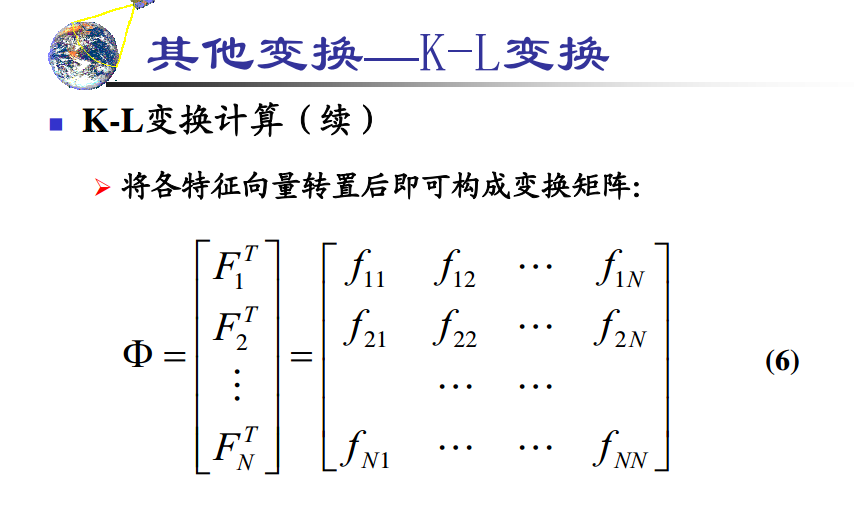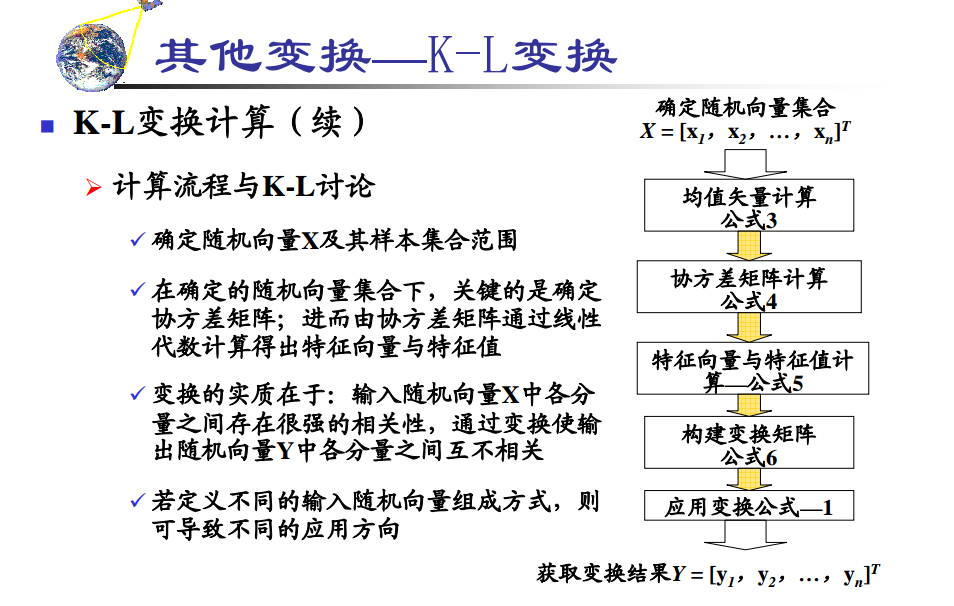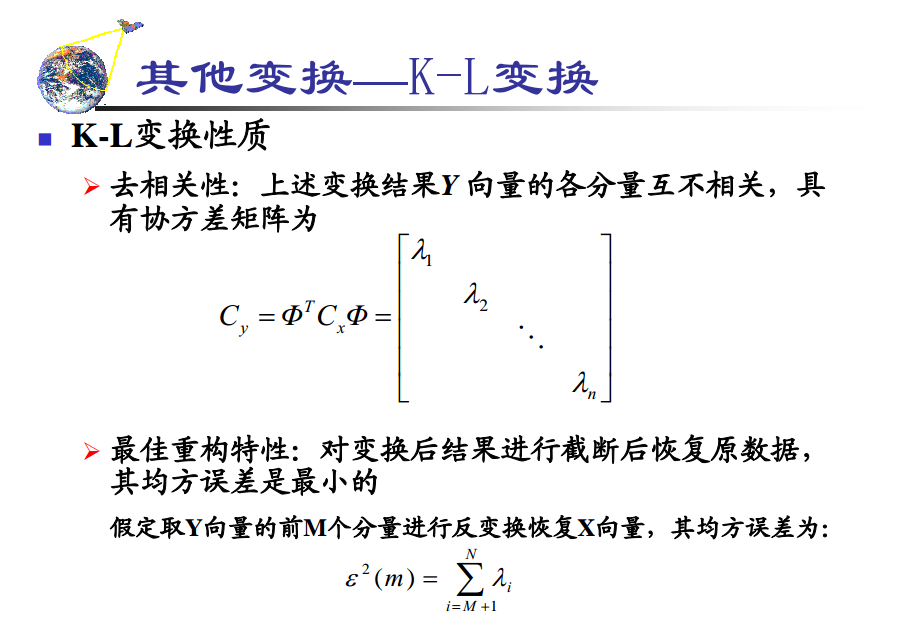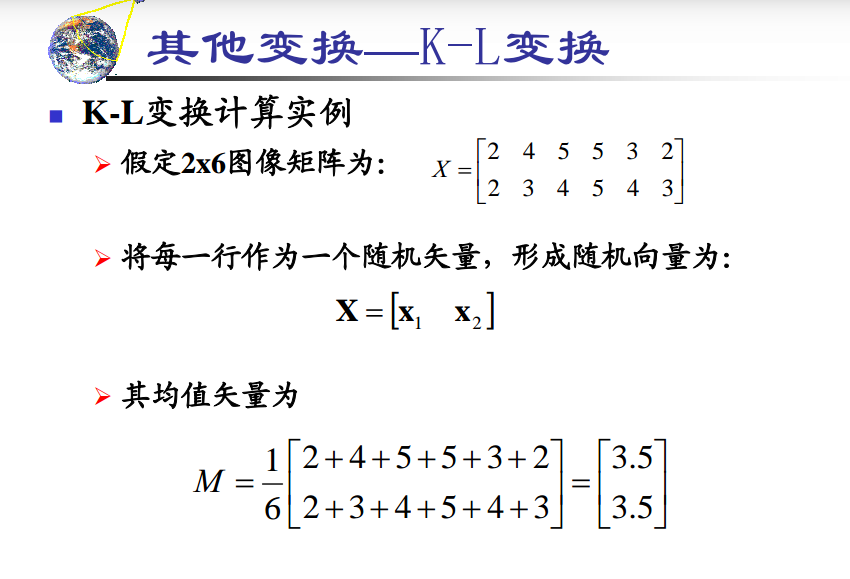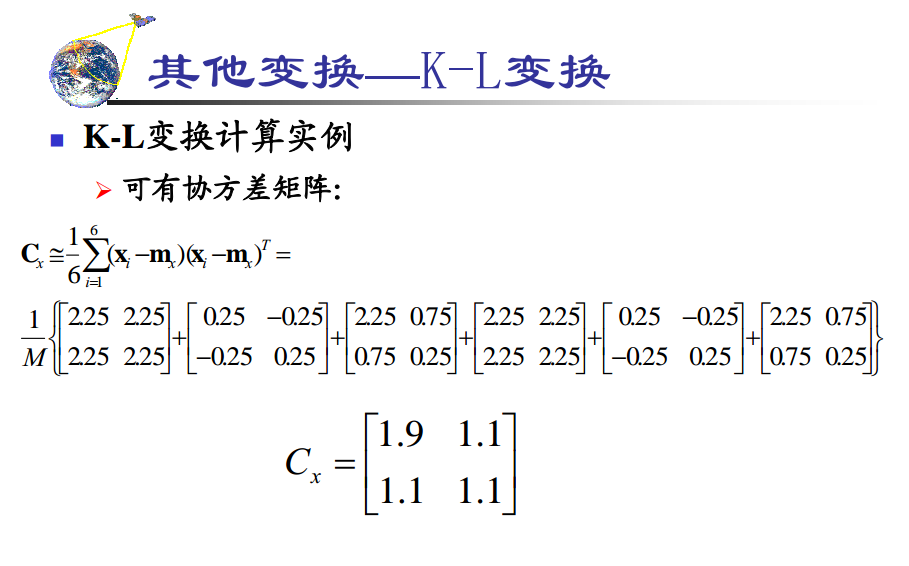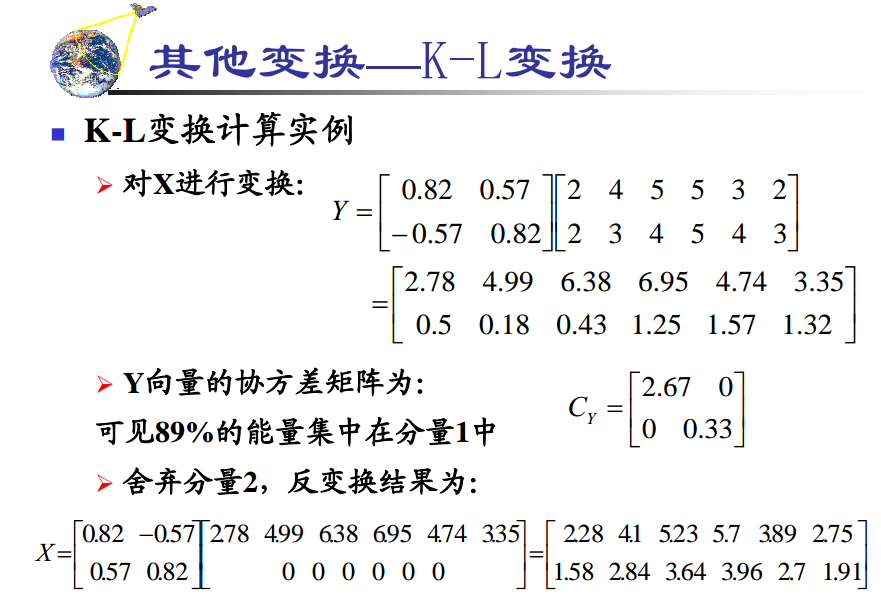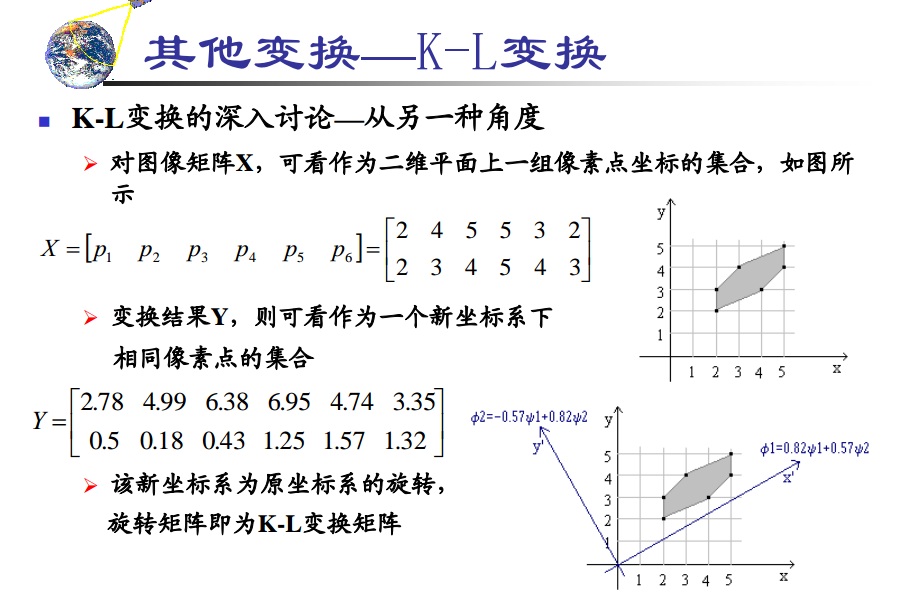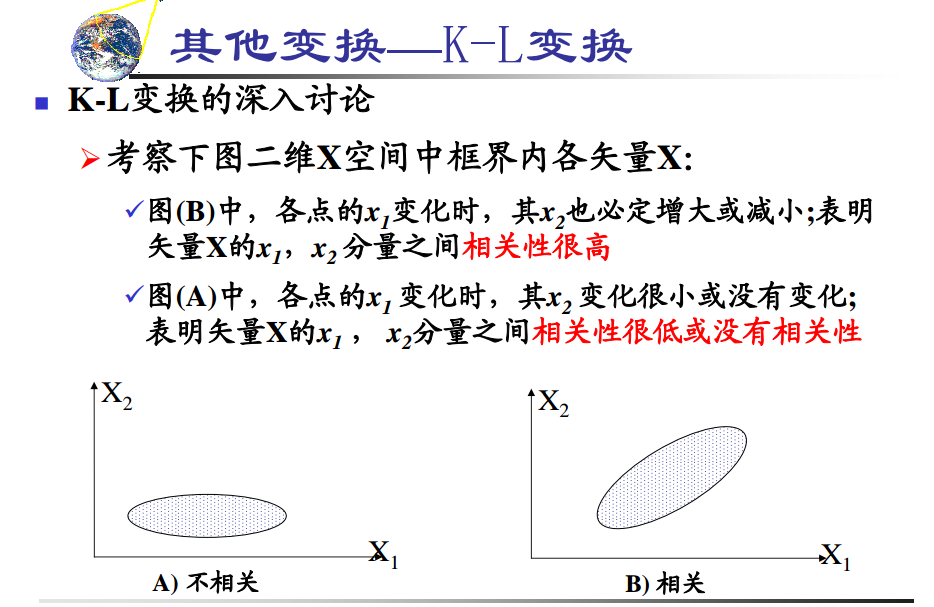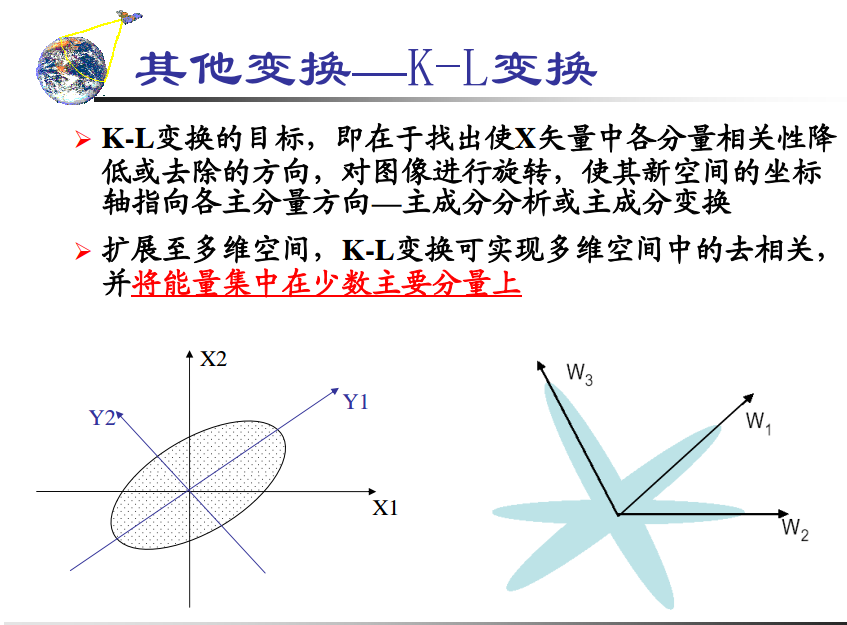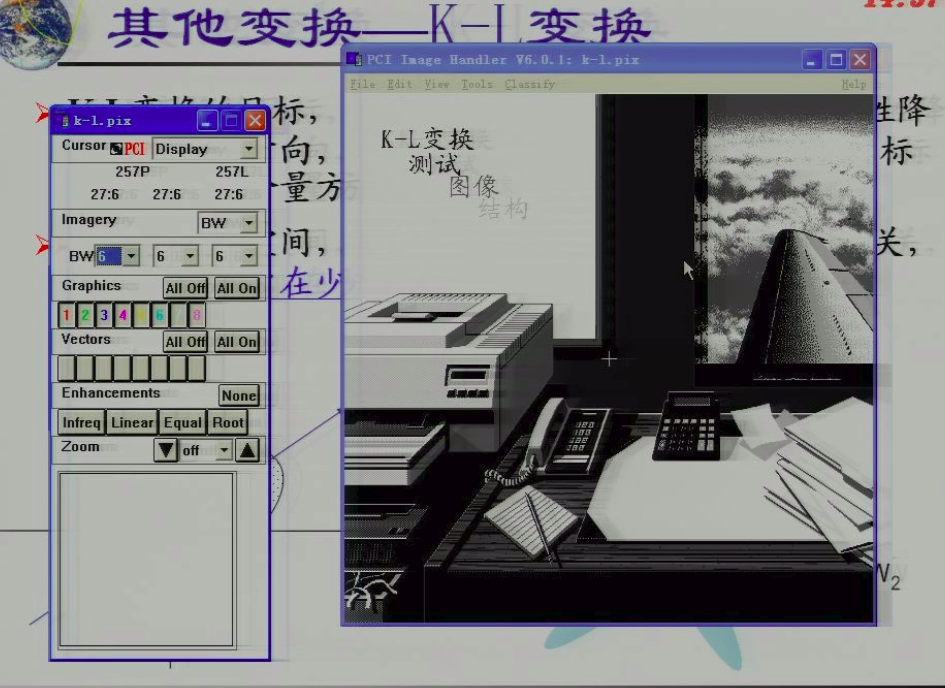### 另外一种比较基础的就是只是在一副图像中运用，使得图像旋转，指向图像的主分量方向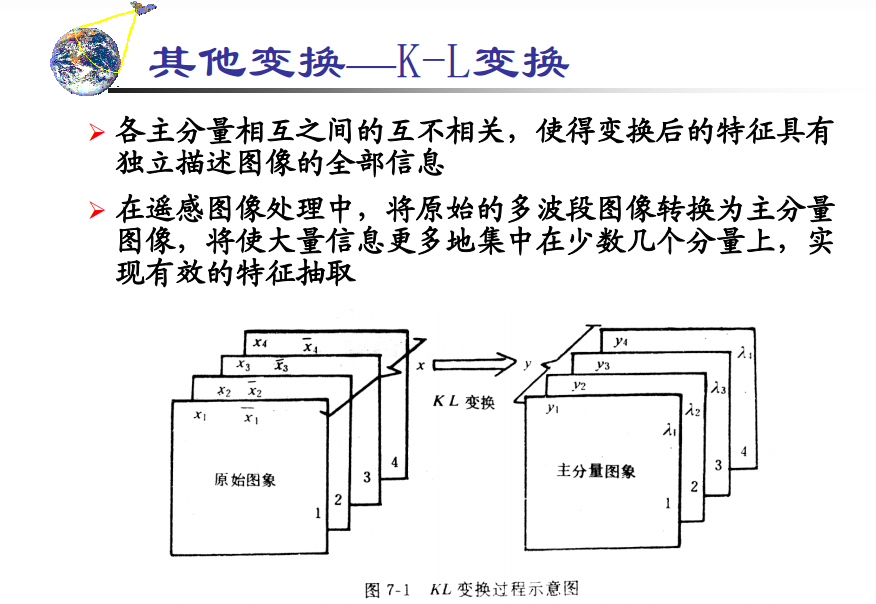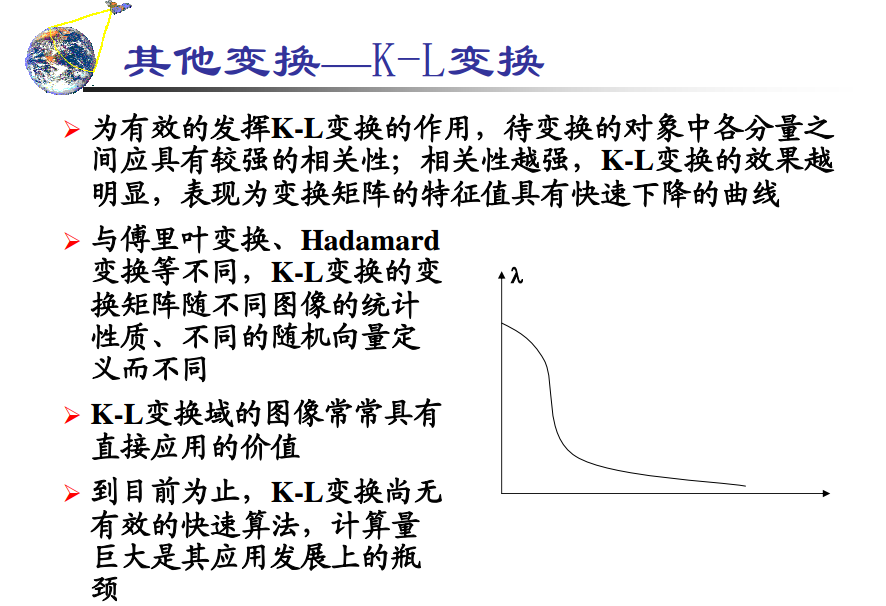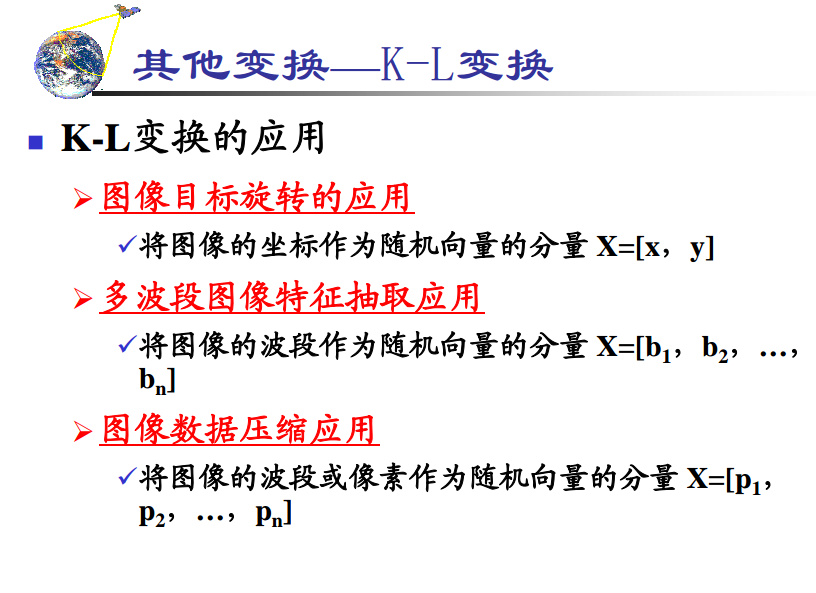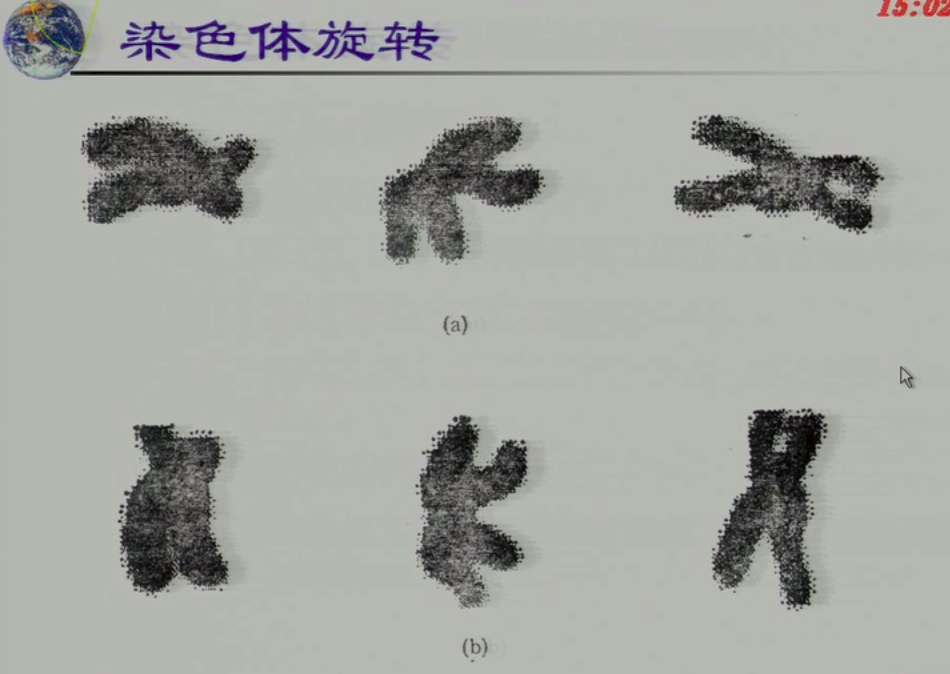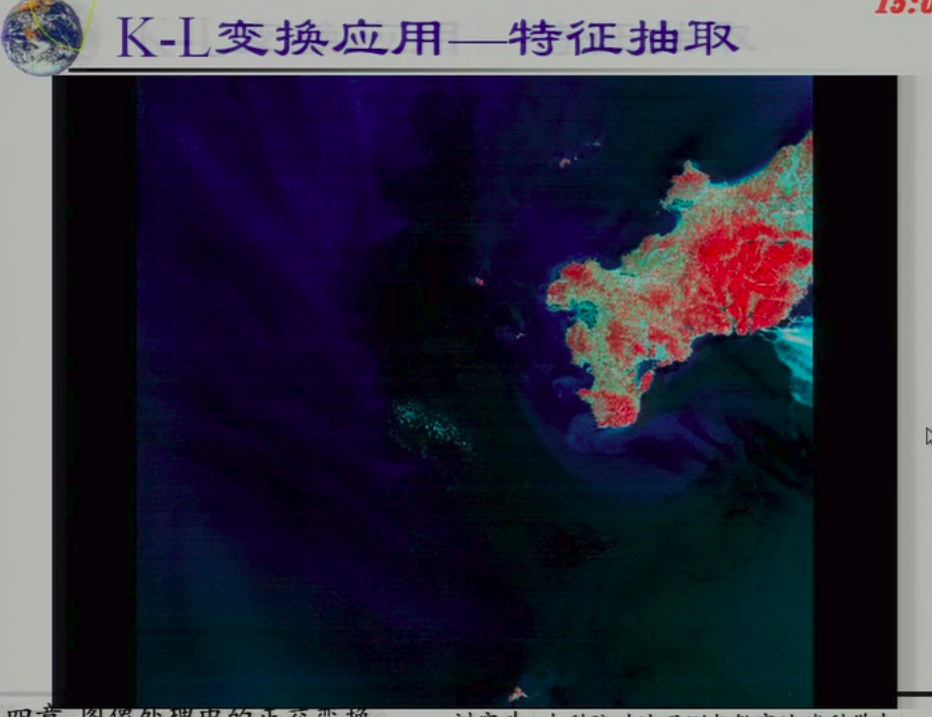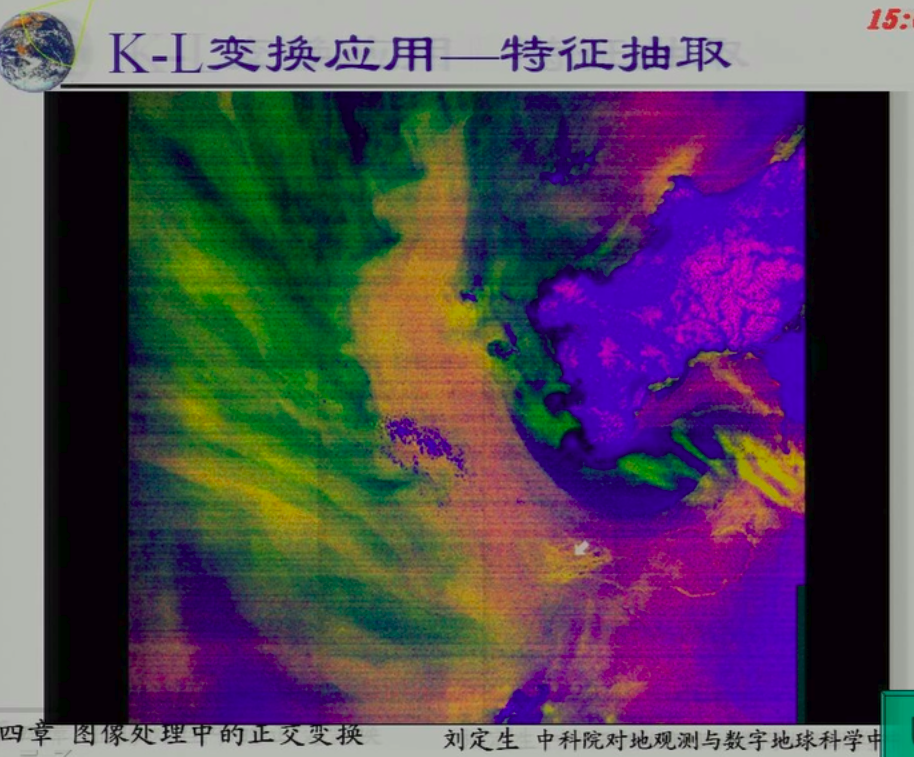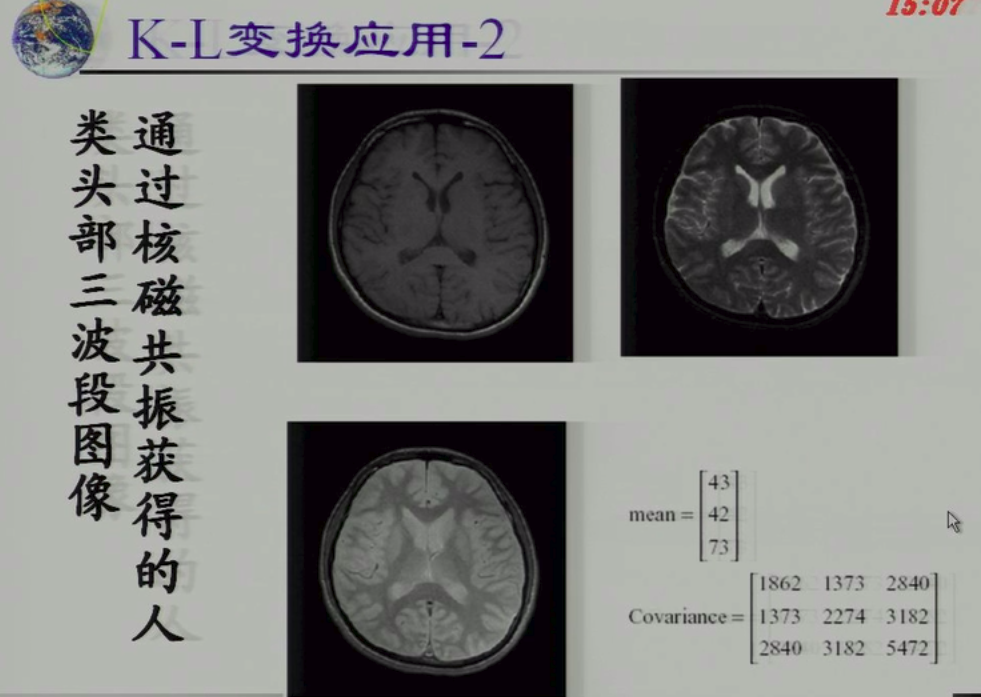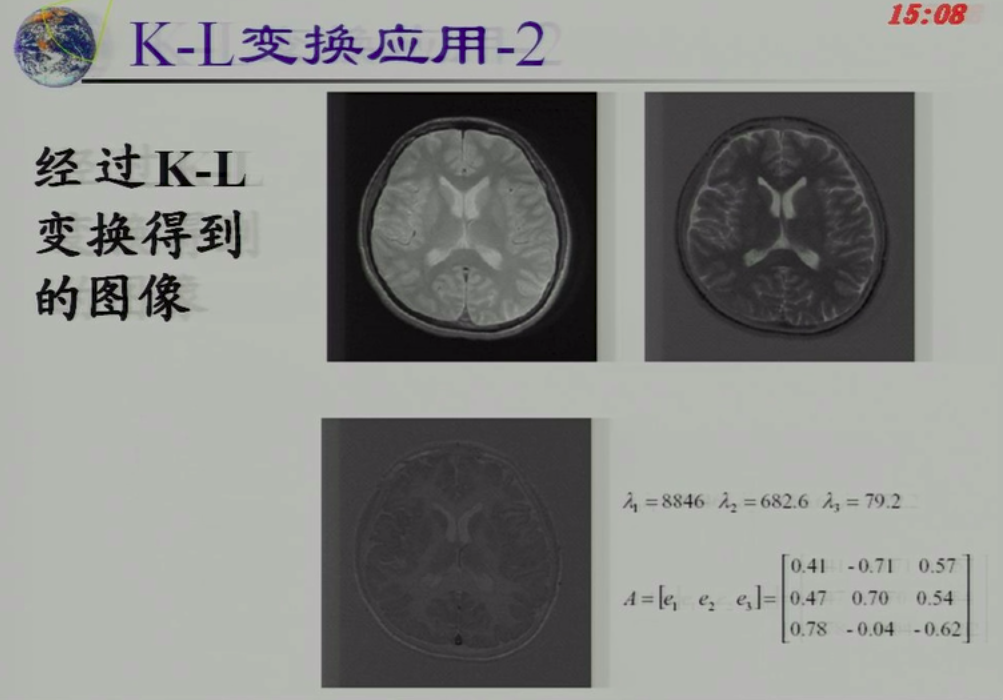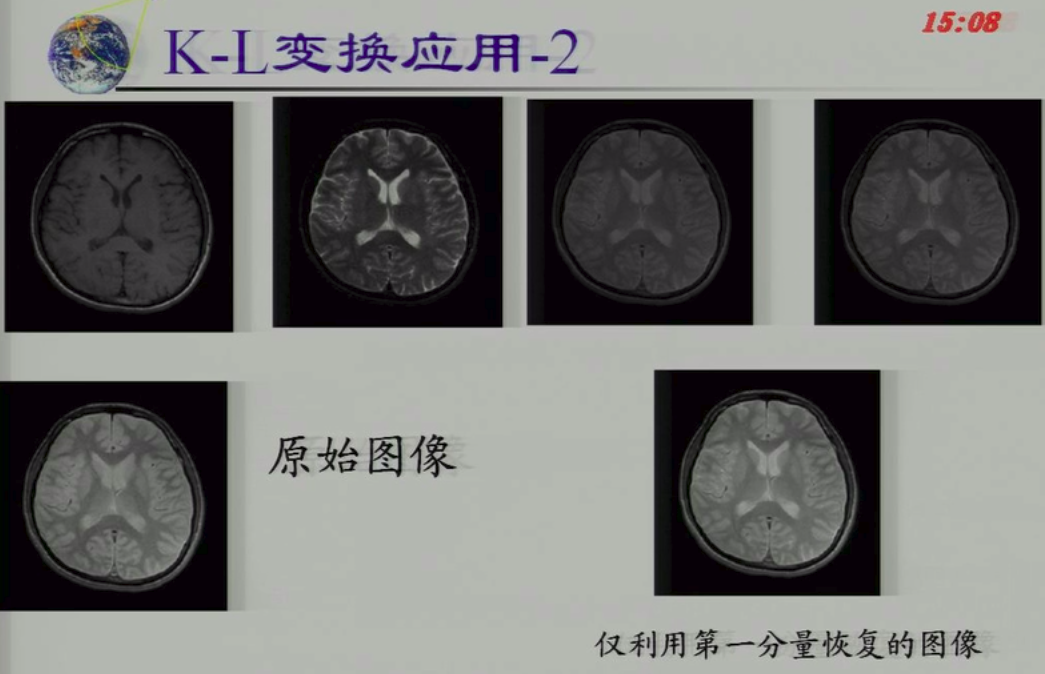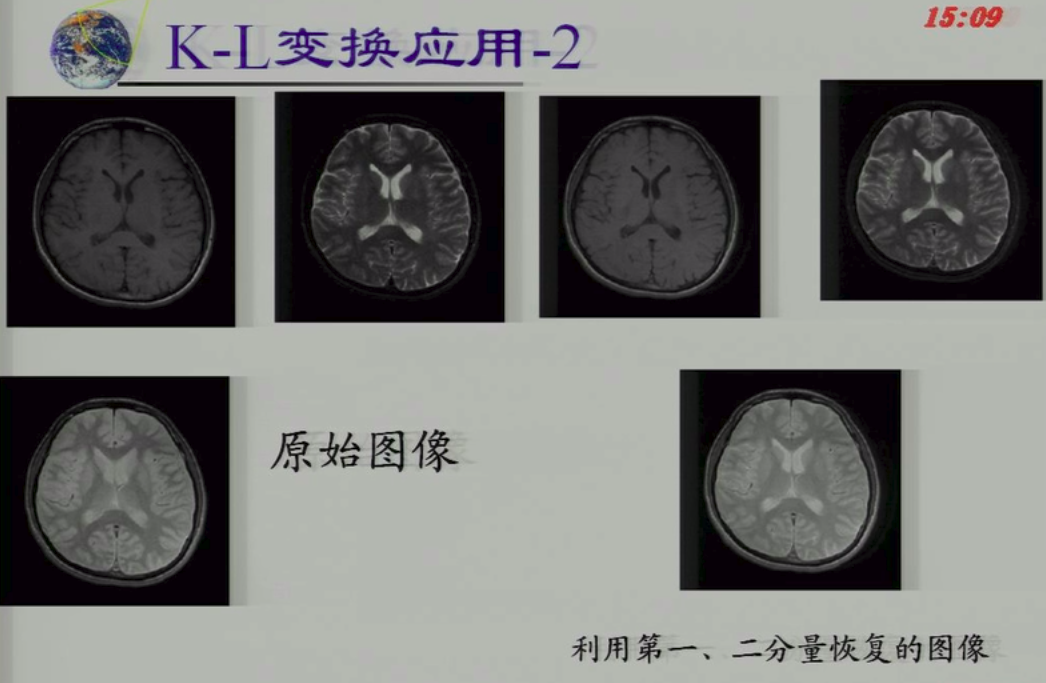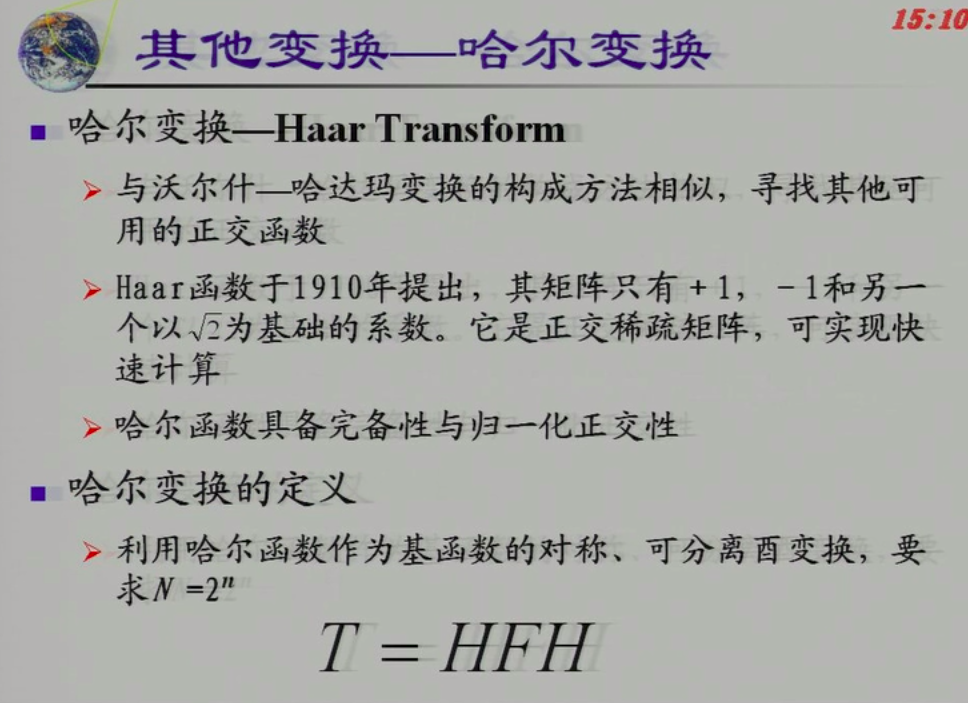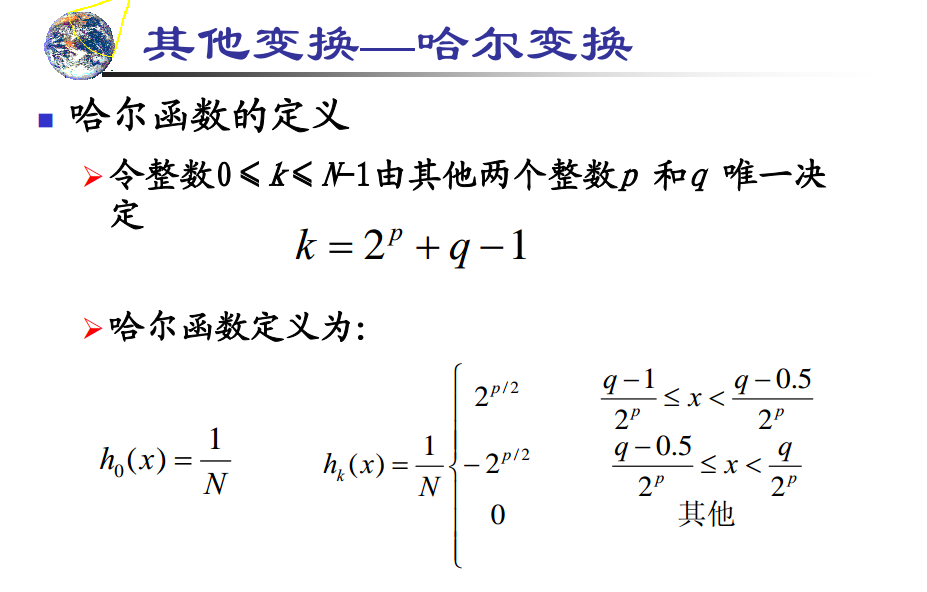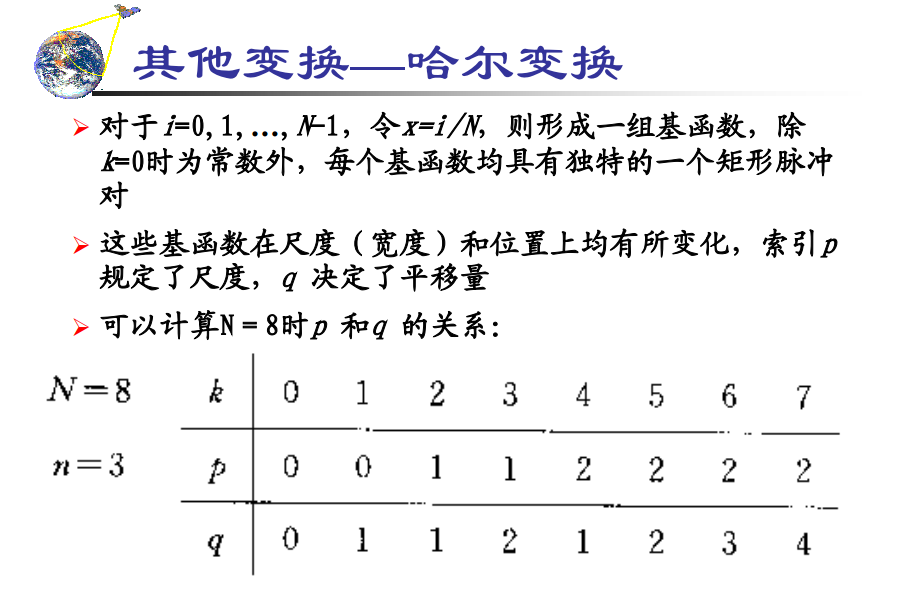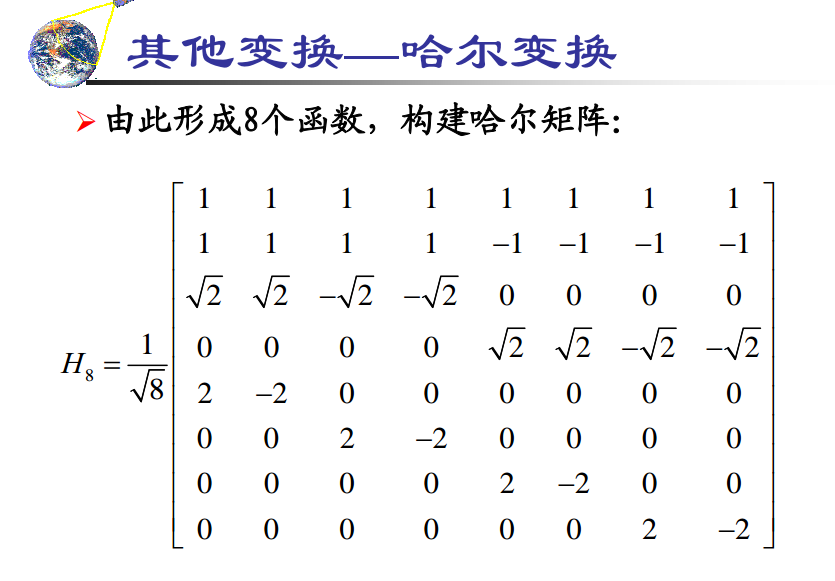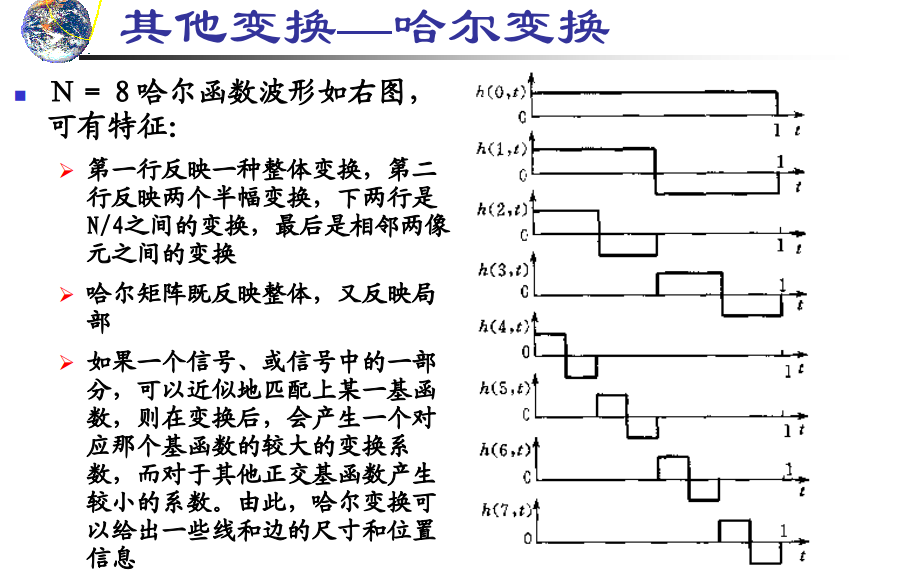### 所以哈尔矩阵既反映局部特征，又反映整体特征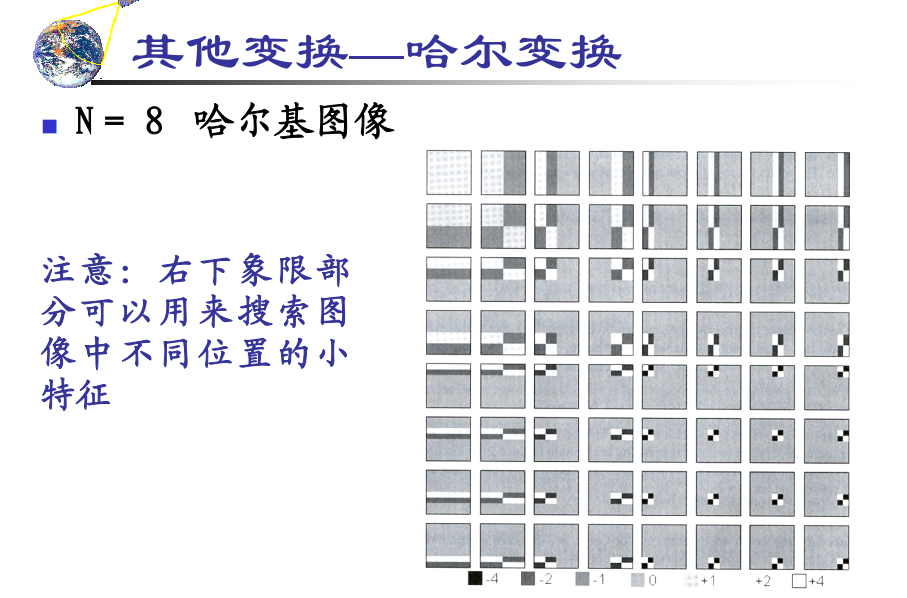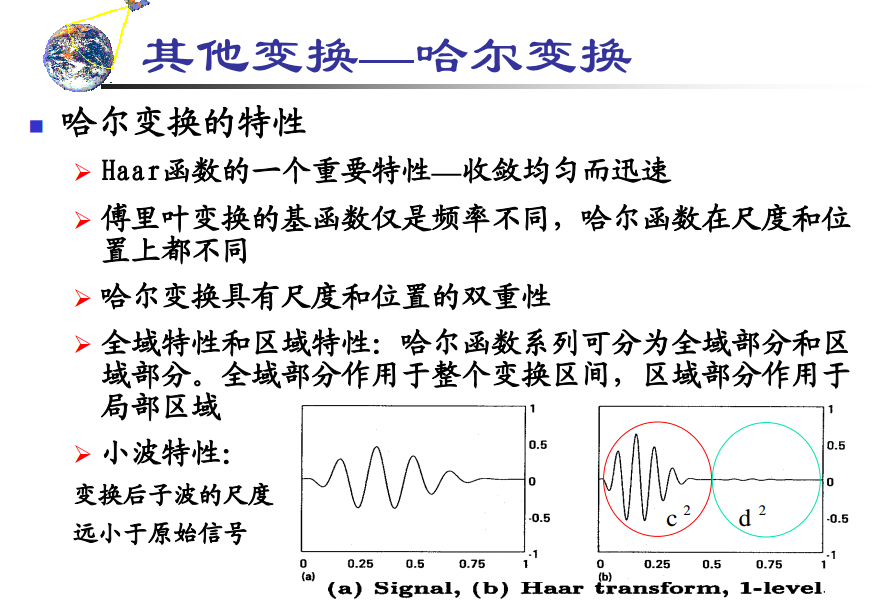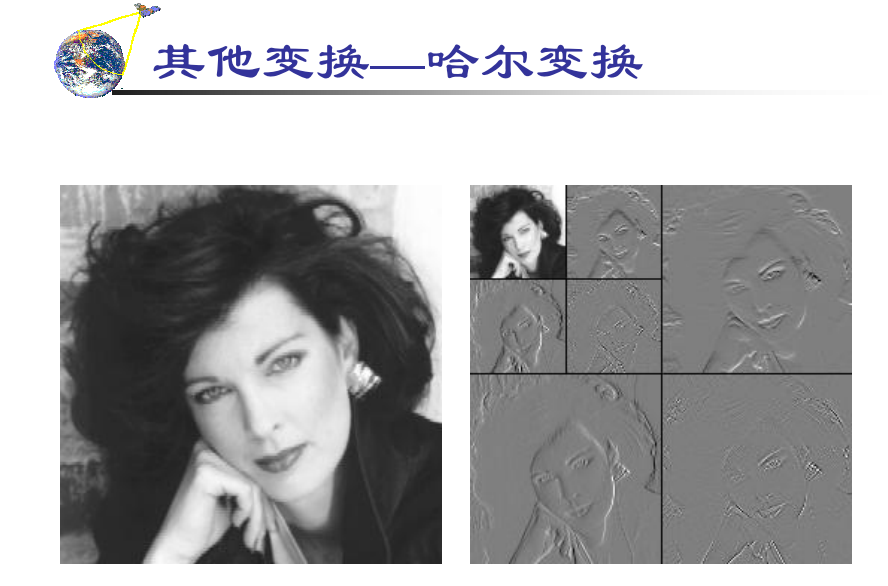2019-07-15 13:04:12 songyuc 阅读数 45
• ###### OpenCV级联分类器训练与使用实战教程

基于OpenCV新版本3.1.0详细讲述了HAAR与LBP级联分类器的基本原理与使用技巧，通过视频中人脸实时检测与眼睛跟踪例子演示了级联分类器在项目中的运用。通过OpenCV自带的训练工具实现样本数据训练生成自己的级联分类器文件，使用它实现自定义对象检测。

1579 人正在学习 去看看 贾志刚

# 2 小波变换介绍

• ###### OpenCV级联分类器训练与使用实战教程

基于OpenCV新版本3.1.0详细讲述了HAAR与LBP级联分类器的基本原理与使用技巧，通过视频中人脸实时检测与眼睛跟踪例子演示了级联分类器在项目中的运用。通过OpenCV自带的训练工具实现样本数据训练生成自己的级联分类器文件，使用它实现自定义对象检测。

1579 人正在学习 去看看 贾志刚

http://media.cs.tsinghua.edu.cn/~ahz/digitalimageprocess/chapter12/chapt12_ahz.htm

Matlab 小波变换

lean图像的行列应该满足2的幂次方

```img  =  imread('lena.jpg');
img =rgb2gray(img);
img = double(img);

[ca1 ch1,cv1,cd1] = dwt2(img,'haar');
figure;
imshow([uint8(ca1),uint8(ch1);uint8(cv1),uint8(cd1)]);
title('first wavelet decomposition');

[ca2 ch2,cv2,cd2] = dwt2(ca1,'haar');
figure;
imshow([uint8(ca2),uint8(ch2);uint8(cv2),uint8(cd2)]);
title('second wavelet decomposition');

% restruct img
img_ca1 =  idwt2(ca2,ch2,cv2,cd2,'haar');
img_re = idwt2(img_ca1,ch1,cv1,cd1,'haar');
figure;
imshow( uint8(img_re));
title('wavelet restruct');```